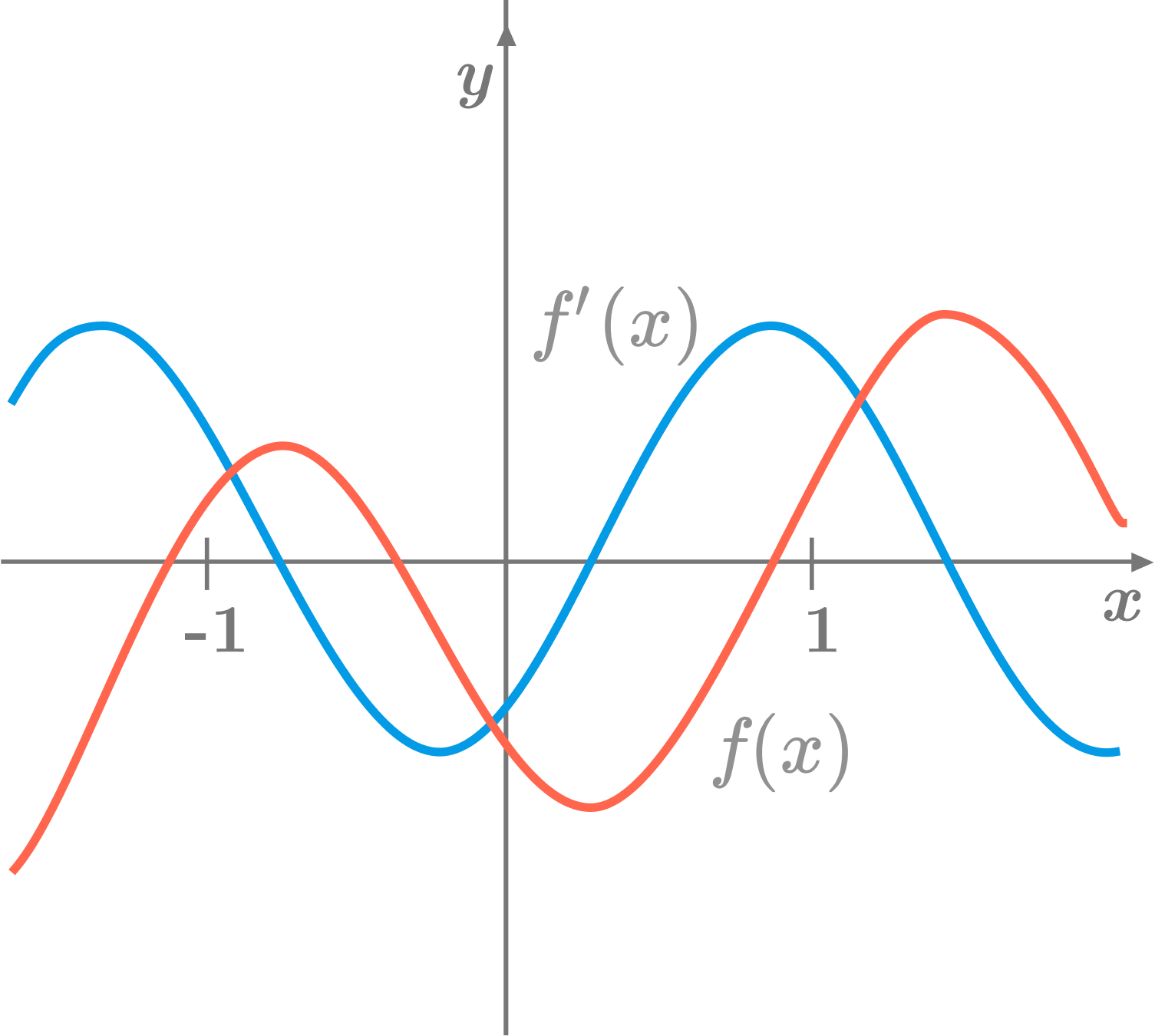# Calculus problem #26933

Calculus Level 1In the above diagram, the red curve is the graph of a function $f,$ and the blue curve is the graph of its first derivative $f'.$ What is the relationship between $f'(-1)$ and $f''(1) ?$

×